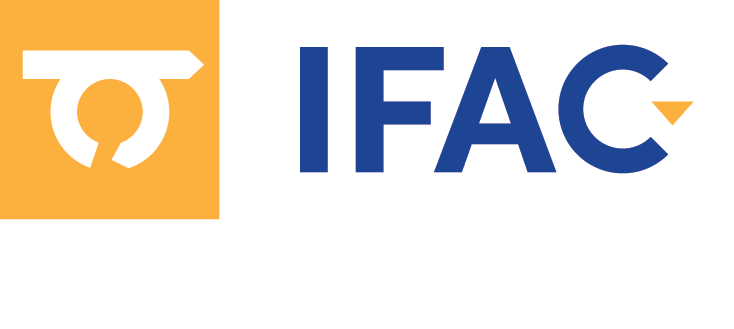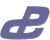3rd IFAC Workshop on Control of Systems Governed by Partial Differential Equations CPDE and XI Workshop Control of Distributed Parameter Systems, CDPS 2019. Oaxaca, Mexico. May 20-24, 2019

### 3rd IFAC/IEEE CSS Workshop on Control of Systems Governed by Partial Differential Equation, XI Workshop Control of Distributed Parameter SystemsMay 20-24, 2019, Oaxaca, Mexico

Last updated on May 27, 2019. This conference program is tentative and subject to change

### Technical Program for Monday May 20, 2019

To show or hide the keywords and abstract of a paper (if available), click on the paper title
Open all abstracts   Close all abstracts
 MoPJ Plenary Session, Oaxaca I Add to My Program Thomas Meurer. Multi-Agent Systems – Continuum Models and PDE-Based Control Chair: Gruene, Lars Univ of Bayreuth
 MoInvaJ Invited Talk, Oaxaca I Add to My Program Miroslav Krstic. Control of Systems of Stefan Type
 MoSJ Invited Session, Oaxaca I Add to My Program Progress in Feedback Control of Partial Differential Equations Chair: Gruene, Lars Univ of Bayreuth Co-Chair: Alla, Alessandro PUC-Rio Organizer: Gruene, Lars Univ of Bayreuth 10:45-11:10, Paper MoSJ.1 Add to My Program Dissipativity-Based Backstepping Boundary Control for a Class of Semilinear Partial Integro-Differential Equations (I) Schaum, Alexander Kiel University Meurer, Thomas Christian-Albrechts-University Kiel Abstract: The problem of state feedback control for a class of semilinear partial integro- differential equations is addressed within the framework of dissipativity theory. Adapting ideas from absolute stability theory the system is viewed as interconnection of a linear dynamic and a nonlinear static subsystem. Using this interpretation sufficient conditions for the stabilization of the zero solution by means of a backstepping state feedback controller are derived considering a class of nonlinearities satisfying a quadratic dissipativity condition. The approach is illustrated using numerical simulations for a representative case example. 11:10-11:35, Paper MoSJ.2 Add to My Program Numerical Verification of Turnpike and Continuity Properties for Time-Varying PDEs (I) Gruene, Lars Univ of Bayreuth Pirkelmann, Simon University of Bayreuth Keywords: Optimal Control, Computational Methods Abstract: To prove approximate closed loop optimality of economic model predictive control of time varying systems, continuity assumptions for the optimal value function and the turnpike property are sufficient conditions. It can be difficult to prove these assumptions analytically for a given example. In this paper we present a numerical approach that aims to verify the assumptions by simulations for a system involving a convection-diffusion equation with boundary control. The results show that the assumptions are realistic and can be met for practical problems. 11:35-12:00, Paper MoSJ.3 Add to My Program On Dissipativity of the Fokker-Planck Equation for the Ornstein-Uhlenbeck Process (I) Fleig, Arthur University of Bayreuth Gruene, Lars Univ of Bayreuth Abstract: We study conditions for stability and near optimal behavior of the closed loop generated by Model Predictive Control for tracking Gaussian probability density functions associated with linear stochastic processes. To this end, we analyze whether the corresponding optimal control problems are strictly dissipative, as this is the key property required to infer such statements when tracking so-called unreachable setpoints. For verifying strict dissipativity, the choice of the so-called storage function is crucial. We focus on linear ones due to their close connection to the Lagrange function. The Ornstein-Uhlenbeck process serves as a prototype for our analysis, in which we show the limits of linear storage functions and present nonlinear alternatives, providing structural insight into dissipativity in case of bilinear system dynamics. 12:00-12:25, Paper MoSJ.4 Add to My Program High-Order Approximation of the Finite Horizon Control Problem Via a Tree Structure Algorithm (I) Alla, Alessandro PUC-Rio Falcone, Maurizio SAPIENZA - Universita' Di Roma Saluzzi, Luca Gran Sasso Science Institute Keywords: Optimal Control Abstract: Solving optimal control problems via Dynamic Programming is a difficult task that suffers for the "curse of dimensionality". This limitation has reduced its practical impact in real world applications since the construction of numerical methods for nonlinear PDEs in very high dimension is practically unfeasible. Recently, we proposed a new numerical method to compute the value function avoiding the construction of a space grid and the need for interpolation techniques. The method is based on a tree structure that mimics the continuous dynamics and allows to solve optimal control problems in high-dimension. This property is particularly useful to attack control problems with PDE constraints. We present a new high-order approximation scheme based on the tree structure and show some numerical results. 12:25-12:50, Paper MoSJ.5 Add to My Program Robust Controller versus Numerical Model Uncertainties for Stabilization of Navier-Stokes Equations (I) Benner, Peter Max Planck Institute for Dynamics of Complex Technical Systems Heiland, Jan Max Planck Institute for Dynamics of Complex Technical Systems Werner, Steffen W. R. Max Planck Institute for Dynamics of Complex Technical Systems Keywords: Flow Control, Computational Methods Abstract: We consider the stabilization of incompressible fluid flow using linearized and spatially discretized models. In order to potentially work in applications, the designed controller must stabilize the discrete model with a robustness margin that covers linearization, discretization, and modeling errors. We expand on previous results that a linearization error in the infinite-dimensional model amounts to a coprime factor uncertainty and show that H-infinity-robust controllers can compensate this in the discrete approximation. In numerical experiments, we quantify the robustness margins and show that the H-infinity-robust controller, unlike the LQG-controller, is capable of stabilizing nonlinear incompressible Navier-Stokes equations with an inexact linearization.
 MoSL Regular Session, Guelaguetza Add to My Program Controllability, Observability and Stability Analysis Chair: Avdonin, Sergei University of Alaska Fairbanks Co-Chair: Macchelli, Alessandro Univ. of Bologna - Italy 10:45-11:10, Paper MoSL.1 Add to My Program Exact Controllability for the Wave Equation on Star Graphs Avdonin, Sergei University of Alaska Fairbanks Zhao, Yuanyuan University of Alaska Fairbanks Abstract: We consider initial boundary value and control problems for the wave equation on tree graphs, where Dirichlet controls are applied at either all or all but one boundary vertices. For a three-edge star graph, we prove the exact shape, velocity and full controllability of the systems. We propose new constructive algorithms solving the initial boundary value problem and the boundary control problem and present a numerical example. 11:10-11:35, Paper MoSL.2 Add to My Program On the Boundary Controllability of Coupled 1-D Wave Equations Park, Jeff University of Alaska Fairbanks Keywords: Optimal Control Abstract: This paper focuses on the exact controllability of a system of N one-dimensional coupled wave equations where the control is applied on a part of the boundary by a single control. In particular, we consider a coupling matrix A with a single eigenvalue of algebraic multiplicity N. We give a Kalman condition (necessary and sufficient) and give a description of the attainable set. In general, this set is not optimal, but can be refined under certain conditions. 11:35-12:00, Paper MoSL.3 Add to My Program A Stability Analysis Based on Dissipativity of Linear and Nonlinear Repetitive Control Califano, Federico University of Twente Macchelli, Alessandro Univ. of Bologna - Italy Abstract: This paper deals with repetitive control (RC). More specifically, a parametrised version of the repetitive compensator, i.e. of the infinite dimensional controller employed in RC schemes, modelled as a boundary control system (BCS) in port-Hamiltonian form is presented. Well-posedness and stability of such control scheme are rigorously addressed thanks to novel tools based on dissipativity theory and originally developed for the stabilisation of BCS. Here, the linear and the nonlinear cases are tackled, and in both the cases the classes of plants for which RC schemes are exponentially stable are determined. Moreover, and explicit motivation of perfect asymptotic tracking and disturbance rejection for exponentially stable RC systems without relying on the internal model theory is provided. Simulations to show the validity of the analysis are reported. 12:00-12:25, Paper MoSL.4 Add to My Program Brayton-Moser Formulation of High-Order Distributed Port-Hamiltonian Systems with One-Dimensional Spatial Domain Macchelli, Alessandro Univ. of Bologna - Italy Abstract: For a class of distributed port-Hamiltonian systems with dissipation characterised by high-order differential operators, one-dimensional domain, and boundary actuation and sensing, an equivalent Brayton-Moser formulation is obtained. The result is that the state evolution is described by a gradient equation with respect to a storage function, the "mixed-potential," that has the dimensions of power. This is the main difference with respect to the port-Hamiltonian form, where the dynamic depends on the derivatives up to a certain order and with respect to the spatial coordinate of the gradient of the Hamiltonian function, i.e. of the total energy.
 MoInvbJ Invited Talk, Oaxaca I Add to My Program Sergei Avdonin. Control, Observation and Identication Problems for the Wave Equation on Metric Graphs 15:00-15:45, Paper MoInvbJ.1 Add to My Program Control, Observation and Identification Problems for the Wave Equation on Metric Graphs Avdonin, Sergei University of Alaska Fairbanks Abstract: We consider control and inverse problems for the wave and Schredinger equations on metric graphs, including graphs with cycles. We find conditions for the exact controllability of the wave equation on graphs. The complete solution of the inverse problem consists of reconstructing the graph's topology, the lengths of all edges and coefficients of the equations. We prove the uniqueness theorem and propose a stable identification algorithm, which is based on the leaf peeling method proposed earlier for graphs without cycles (trees).
 MoInvcJ Invited Talk, Oaxaca I Add to My Program Héctor Ramírez Estay. Some Recent Results on Boundary Control of Infinite Dimensional Port-Hamiltonian Systems on 1-D Spatial Domains
 MoInvdJ Invited Talk, Oaxaca I Add to My Program Yann Le Gorrec. Control by Interconnection and Energy Shaping of Infinite Dimensional Port Hamiltonian SystemsThis site is protected by copyright and trademark laws under US and International law.